# Hyper-netted chain

The hyper-netted chain (HNC) equation has a clear physical basis in the Kirkwood superposition approximation (Ref. 1). The hyper-netted chain approximation is obtained by omitting the elementary clusters,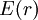$E(r)$, in the exact convolution equation for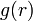$g(r)$. The hyper-netted chain approximation was developed almost simultaneously by various groups, namely: van Leeuwen, Groeneveld and de Boer, 1959 (Ref. 2). Morita and Hiroike, 1960 (Ref.s 3-8), Rushbrooke, 1960 (Ref. 9), Verlet, 1960 (Ref. 10), and Meeron, 1960 (Ref. 11). The hyper-netted chain omits the bridge function, i.e.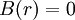$B(r) =0$, thus the cavity correlation function becomes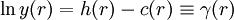$\ln y (r) = h(r) -c(r) \equiv \gamma (r)$

The hyper-netted chain closure relation can be written as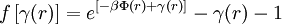$f \left[ \gamma (r) \right] = e^{[-\beta \Phi (r) + \gamma (r)]} - \gamma (r) -1$

or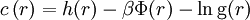$c\left(r\right)= h(r) - \beta \Phi(r) - \ln {\rm g}(r)$

or (Eq. 12 Ref. 1)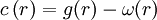$c\left( r \right)= g(r) - \omega(r)$

where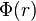$\Phi(r)$ is the intermolecular pair potential. The hyper-netted chain approximation is well suited for long-range potentials, and in particular, Coulombic systems. For details of the numerical solution of the hyper-netted chain equation for ionic systems (see Ref. 12).